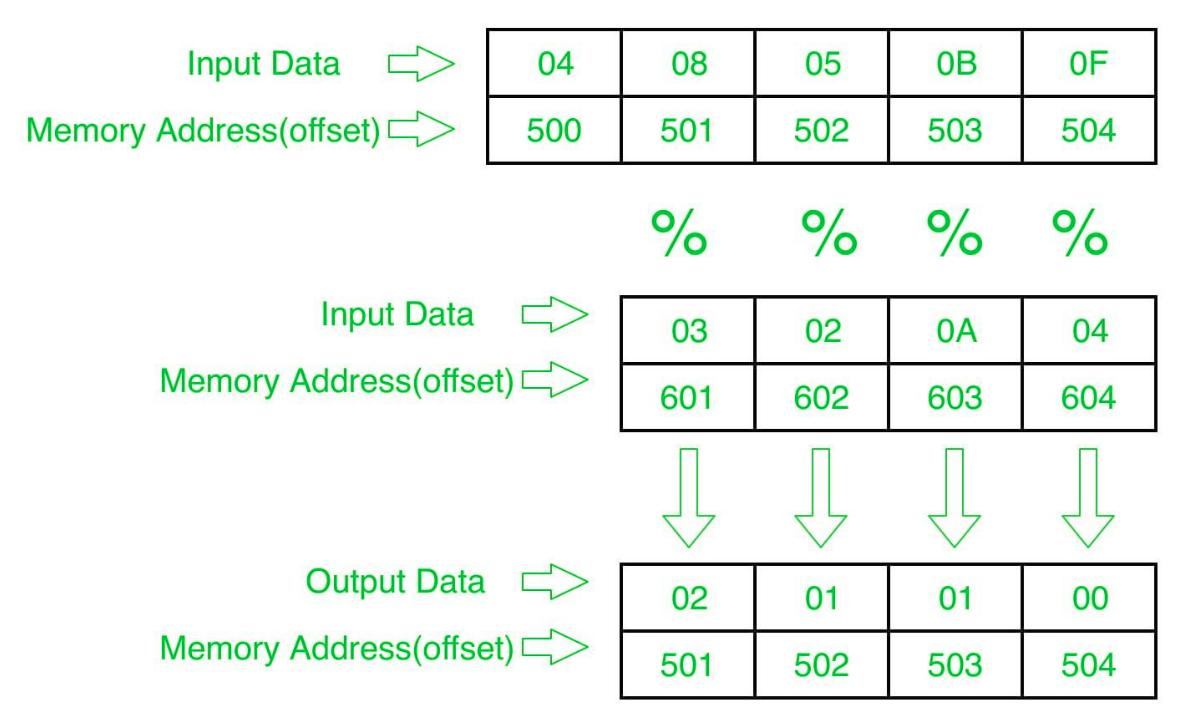# 8086 program to determine modulus of first array elements corresponding to another array elements

Problem – Write a program in 8086 microprocessor to determine modulus of corresponding 8 bit n elements of first array with 8-bit n numbers of second array, where size “n” is stored at offset 500 and the numbers of first array are stored from offset 501 and the numbers of second array are stored from offset 601 and store the result numbers into first array i.e offset 501.

Example –Algorithm –

1. Store 500 to SI and 601 to DI and Load data from offset 500 to register CL and set register CH to 00 (for count).
2. Increase the value of SI by 1.
3. Load first number(value) from next offset (i.e 501) to register AL.
4. store 00 at register AH.
5. Divide the value in register AX by value at offset DI.
6. Store the result (value of register AH ) to memory offset SI.
7. Increase the value of SI by 1.
8. Increase the value of DI by 1.
9. Loop above 6 till register CX gets 0.

Program –

400 MOV SI, 500 SI<-500
403 MOV CL, [SI] CL<-[SI]
405 MOV CH, 00 CH<-00
407 INC SI SI<-SI+1
408 MOV DI, 601 DI<-601
40B MOV AL, [SI] AL<-[SI]
40D MOV AH, 00 AH<-00
40F DIV [DI] AX=AX/[DI]
411 MOV [SI], AH AH->[SI]
413 INC SI SI<-SI+1
414 INC DI DI<-DI+1
417 HLT end

Explanation –

1. MOV SI, 500: set the value of SI to 500
2. MOV CL, [SI]: load data from offset SI to register CL
3. MOV CH, 00: set value of register CH to 00
4. INC SI: increase value of SI by 1.
5. MOV DI, 600: set the value of DI to 600.
6. MOV AL, [SI]: load value from offset SI to register AL
7. MOV AH, 00: set value of register AH to 00.
8. DIV [DI]: Divide value of register AX by content at offset DI.
9. MOV [SI], AH: store value of register AH at offset SI.
10. INC SI: increase value of SI by 1.
11. INC DI: increase value of DI by 1.